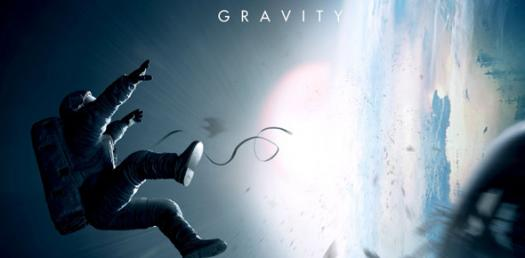# Gravity, Weight, Mass, And Velocity Quiz

5 Questions | Attempts: 592
ShareSettingsThis quiz will be on the lesson plan and experiement preformed in class today.

• 1.
Gravity is exerted on all objects on our planet?
• A.

True

• B.

False

• 2.
The moon has less amounts of gravity than the earth does?
• A.

True

• B.

False

• 3.
Suppose the gravitational force is 10 N between two objects. If the distance between the masses is cut in half, what is the new force between the objects?
• A.

10 N

• B.

20 N

• C.

40 N

• D.

30 N

• 4.
Velocity Equation - V = (__) x t
• 5.
What was the crazy science instructors name in the video we watched in class
• A.

Ming Zhang

• B.

David Coors

• C.

Bill Nye

• D.

Magic Johnson

## Related TopicsBack to top
×

Wait!
Here's an interesting quiz for you.